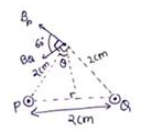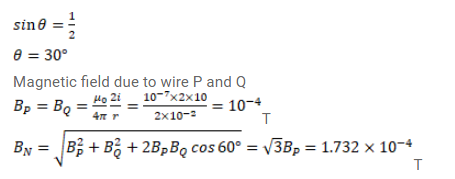# Two parallel wires carry equal currents of

Question:

Two parallel wires carry equal currents of $10 \mathrm{~A}$ along the same direction and are separated by a distance of $2.0 \mathrm{~cm}$. Find the magnetic field at a point which is $2.0 \mathrm{~cm}$ away from each of these wires.

Solution: